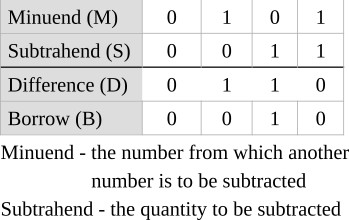Home   >   Textbooks   >   Basic Electronics   >   Combinational Logic   >   Binary Subtractor   >

Combinational Logic

# Binary Subtractor

A binary subtractor is a device that accepts two binary numbers at its input and produces a difference at its output.

## Binary Subtraction

A truth table representing the conditions arising from the subtraction of 2 binary digits (M - S) is shown in the table below.As was true with addition of binary digits, more than 1 binary column is usually involved in the arithmetic process. Thus, the table above although valid, is very limited. When more than one column of digits containing minuend and subtrahend is to be subtracted, each digit of the minuend is decreased by the amount of the subtrahend digit in that column, and if the minuend is reduced to a value less than 0 in the process, the minuend digit of the next higher order must be reduced by 1 - the process by which the borrow is obtained.

The table above is also useful in a process called "half-subtraction" which does not take into account a borrow from a previous order.

## Half Subtractor

The rules of operation for the half subtractor are presented in the table above. The difference digit is exactly the same for M minus S as it is for S minus M. The difference for each combination is also the same as the sum digit which appears in the truth table of the half adder. It follows that the logic diagram for the half subtractor is similar to that for the half adder. The only difference is the generation of the correct borrow instead of a carry.

The borrow we will need can be described as "S AND NOT M". Therefore, the half subtractor in the figure below can be assembled. The difference of the inputs is produced by the quarter adder (XOR gate); the borrow is produced by an added NOT-AND gate circuit.Half subtractor.

## Full Subtractor

Subtraction, like addition, can be performed in two steps if two-input circuits (half subtractors) are used. The figure below shows one way in which two half subtractors can be interconnected to form a full subtractor. Like the full adder, this circuit can be repeated in a chain to form a cascaded subtractor.Full subtractor.